Center of mass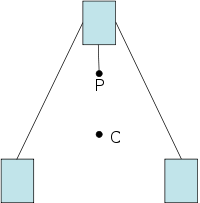Diagram of an educational toy that balances on a point: the CM (C) settles below its support (P). Any object whose CM is below the fulcrum will not topple.

In physics, the center of mass (CM) of a system of particles is a specific point at which the system's mass behaves (for many purposes) as if it were concentrated. The center of mass is a function of only the positions and masses of the particles that make up the system. In the context of an entirely uniform gravitational field, the center of mass is often called the center of gravity—the point where gravity can be said to act. By locating the center of mass of a system, one can analyze the motion of the entire system as distinguished from the motion of its individual parts.

In the case of a rigid body, the position of its center of mass is fixed in relation to the object (but not necessarily in contact with it). In the case of a loose distribution of masses in free space, such as, say, shot from a shotgun, the position of the center of mass is a point in space among them that may not correspond to the position of any individual mass.

The center of mass of a body does not always coincide with its intuitive geometric center. For example, engineers try to design a sport car such that its center of gravity is as low as possible, to make the car handle better. When a high jumper performs a "Fosbury Flop," it involves bending the body in such a way that the jumper can clear the bar while his or her center of mass does not. Also, an object will not topple as long as its center of mass lies within its base of support.

The so-called "center of gravity frame" (a less-preferred term for the center of momentum frame) is an inertial frame in which the center of mass of the system is at rest.

Examples

• The center of mass of a two-particle system lies on the line connecting the particles (or, more precisely, their individual centers of mass). The center of mass is closer to the more massive object. (For details, see barycenter below.)
• The center of mass of a ring is at the center of the ring (in the air).
• The center of mass of a solid triangle lies on all three medians and therefore at the centroid, which is also the average of the three vertices.
• The center of mass of a rectangle is at the intersection of the two diagonals.
• In a spherically symmetric body, the center of mass is at the center. This approximately applies to the Earth: The density varies considerably, but it mainly depends on depth and less on the other two coordinates.
• More generally, for any symmetry of a body, its center of mass will be a fixed point of that symmetry.

History

The concept of center of gravity was first introduced by the ancient Greek mathematician, physicist, and engineer Archimedes of Syracuse. Archimedes showed that the torque exerted on a lever by weights resting at various points along the lever is the same as what it would be if all of the weights were moved to a single point—their center of gravity. In work on floating bodies he demonstrated that the orientation of a floating object is the one that makes its center of gravity as low as possible. He developed mathematical techniques for finding the centers of gravity of objects of uniform density of various well-defined shapes, in particular a triangle, a hemisphere, and a frustum of a circular paraboloid.

In the Middle Ages, theories on the center of gravity were further developed by Abū Rayhān al-Bīrūnī, al-Razi (Latinized as Rhazes), Omar Khayyám, and al-Khazini.

Mathematical definition

The center of mass$\mathbf{R}$ of a system of particles is defined as the average of their positions,$\mathbf{r}_i$, weighted by their masses,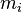$m_i$: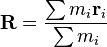$\mathbf{R} = { \sum m_i \mathbf{r}_i \over \sum m_i }$

For a continuous distribution with mass density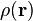$\rho(\mathbf{r})$ and total mass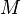$M$, the sum becomes an integral:$\mathbf R =\frac 1M \int \mathbf{r} \; dm = \frac 1M \int\rho(\mathbf{r})\, \mathbf{r} \ dV =\frac{\int\rho(\mathbf{r})\, \mathbf{r} \ dV}{\int\rho(\mathbf{r})\ dV}$

If an object has uniform density, then its center of mass is the same as the centroid of its shape.

Derivation

The following equations of motion assume that there is a system of particles governed by internal and external forces. An internal force is a force caused by the interaction of the particles within the system. An external force is a force that originates from outside the system, and acts on one or more particles within the system. The external force need not be due to a uniform field.

For any system with no external forces, the center of mass moves with constant velocity. This applies for all systems with classical internal forces, including magnetic fields, electric fields, chemical reactions, and so on. More formally, this is true for any internal forces that satisfy the weak form of Newton's Third Law.

The total momentum for any system of particles is given by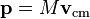$\mathbf{p}=M\mathbf{v}_\mathrm{cm}$

Where M indicates the total mass, and vcm is the velocity of the center of mass. This velocity can be computed by taking the time derivative of the position of the center of mass.

An analogue to Newton's Second Law is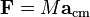$\mathbf{F} = M\mathbf{a}_\mathrm{cm}$

Where F indicates the sum of all external forces on the system, and acm indicates the acceleration of the center of mass.

Letting the total internal force of the system.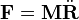$\mathbf{F}=\mathbf{M}\ddot\mathbf{R}$

where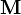$\mathbf{M}$ is the total mass of the system and$\mathbf{R}$ is a vector yet to be defined, since: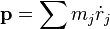$\mathbf{p} = \sum{m_j\dot{r}_j}$

and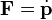$\mathbf{F}=\dot\mathbf{p}$

then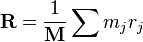$\mathbf{R}=\frac{1}{\mathbf{M}}\sum{m_jr_j}$

Therefore, it yields a vectorial definition for center of mass in terms of the total forces in the system. This is particularly useful for two-body systems.

Rotation and centers of gravity

The center of mass is often called the center of gravity because any uniform gravitational field g acts on a system as if the mass M of the system were concentrated at the center of mass R. This is seen in at least two ways:

• The gravitational potential energy of a system is equal to the potential energy of a point particle having the same mass M located at R.
• The gravitational torque on a system equals the torque of a force Mg acting at R: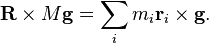$\mathbf{R} \times M\mathbf{g}=\sum_im_i \mathbf{r}_i \times \mathbf{g}.$

If the gravitational field acting on a body is not uniform, then the center of mass does not necessarily exhibit these convenient properties concerning gravity. As the situation is put in Feynman's influential textbook The Feynman Lectures on Physics:

The center of mass is sometimes called the center of gravity, for the reason that, in many cases, gravity may be considered uniform. …In case the object is so large that the nonparallelism of the gravitational forces is significant, then the center where one must apply the balancing force is not simple to describe, and it departs slightly from the center of mass. That is why one must distinguish between the center of mass and the center of gravity.

Later authors are often less careful, stating that when gravity is not uniform, "the center of gravity" departs from the CM. This usage seems to imply a well-defined "center of gravity" concept for non-uniform fields, but there is no such thing. Symon, in his textbook, Mechanics, shows that the center of gravity of an extended body must always be defined relative to an external point, at which location resides a point mass that is exerting a gravitational force on the object in question. Even worse, as Symon says:

For two extended bodies, no unique centers of gravity can in general be defined, even relative to each other, except in special cases, as when the bodies are far apart, or when one of them is a sphere… The general problem of determining the gravitational forces between bodies is usually best treated by means of the concepts of the field theory of gravitation….

Even when considering tidal forces on planets, it is sufficient to use centers of mass to find the overall motion. In practice, for non-uniform fields, one simply does not speak of a "center of gravity."

Aeronautical significance

The center of mass is an important point on an aircraft, which significantly affects the stability of the aircraft. To ensure the aircraft is safe to fly, it is critical that the center of gravity fall within specified limits. This range varies by aircraft, but as a rule of thumb it is centered about a point one quarter of the way from the wing leading edge to the wing trailing edge (the quarter chord point). If the center of mass is ahead of the forward limit, the aircraft will be less maneuverable, possibly to the point of being unable to rotate for takeoff or flare for landing. If the center of mass is behind the aft limit, the moment arm of the elevator is reduced, which makes it more difficult to recover from a stalled condition. The aircraft will be more maneuverable, but also less stable, and possibly so unstable that it is impossible to fly.

Barycenter in astronomy

The barycenter (or barycentre; from the Greek βαρύκεντρον) is the point between two objects where they balance each other. In other words, it is the center of gravity where two or more celestial bodies orbit each other. When a moon orbits a planet, or a planet orbits a star, both bodies are actually orbiting around a point that lies outside the center of the greater body. For example, the moon does not orbit the exact center of the earth, instead orbiting a point outside the earth's center (but well below the surface of the Earth) where their respective masses balance each other. The barycenter is one of the foci of the elliptical orbit of each body. This is an important concept in the fields of astronomy, astrophysics, and the like.

In a simple two-body case, r1, the distance from the center of the first body to the barycenter is given by: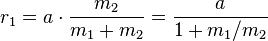$r_1 = a \cdot {m_2 \over m_1 + m_2} = {a \over 1 + m_1/m_2}$

where:

a is the distance between the two bodies' centers;
m1 and m2 are the masses of the two bodies.

r1 is essentially the semi-major axis of the first body's orbit around the barycenter—and r2 = a - r1 the semi-major axis of the second body's orbit. Where the barycenter is located within the more massive body, that body will appear to "wobble" rather than following a discernible orbit.

The following table sets out some examples from our solar system. Figures are given rounded to three significant figures. The last two columns show R1, the radius of the first (more massive) body, and r1/R1, the ratio of the distance to the barycenter and that radius: A value less than one shows that the barycenter lies inside the first body.

Examples
Larger
body
m1
(mE=1)
Smaller
body
m2
(mE=1)
a
(km)
r1
(km)
R1
(km)
r1/R1
Remarks
Earth 1 Moon 0.0123 384,000 4,670 6,380 0.732
The Earth has a perceptible "wobble."
Pluto 0.0021 Charon 0.000,254
(0.121 mPluto)
19,600 2,110 1,150 1.83
Both bodies have distinct orbits around the barycenter, and as such Pluto and Charon were considered as a double planet by many before the redefinition of planet in August 2006.
Sun 333,000 Earth 1 150,000,000
(1 AU)
449 696,000 0.000,646
The Sun's wobble is barely perceptible.
Sun 333,000 Jupiter 318 778,000,000
(5.20 AU)
742,000 696,000 1.07
The Sun orbits a barycenter just above its surface.

If m1 >> m2—which is true for the Sun and any planet—then the ratio r1/R1 approximates to: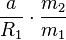${a \over R_1} \cdot {m_2 \over m_1}$

Hence, the barycenter of the Sun-planet system will lie outside the Sun only if: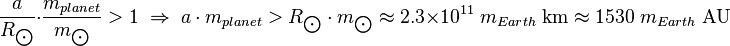${a \over R_{\bigodot}} \cdot {m_{planet} \over m_{\bigodot}} > 1 \; \Rightarrow \; {a \cdot m_{planet}} > {R_{\bigodot} \cdot m_{\bigodot}} \approx 2.3 \times 10^{11} \; m_{Earth} \; \mbox{km} \approx 1530 \; m_{Earth} \; \mbox{AU}$

That is, where the planet is heavy and far from the Sun.

If Jupiter had Mercury's orbit (57,900,000 km, 0.387 AU), the Sun-Jupiter barycenter would be only 5,500 km from the center of the Sun (r1/R1 ~ 0.08). But even if the Earth had Eris' orbit (68 AU), the Sun-Earth barycenter would still be within the Sun (just over 30,000 km from the center).

To calculate the actual motion of the Sun, you would need to sum all the influences from all the planets, comets, asteroids, and so on, of the solar system (see n-body problem). If all the planets were aligned on the same side of the Sun, the combined center of mass would lie about 500,000 km above the Sun's surface.

The calculations above are based on the mean distance between the bodies and yield the mean value r1. But all celestial orbits are elliptical, and the distance between the bodies varies between the apses, depending on the eccentricity, e. Hence, the position of the barycenter varies too, and it is possible in some systems for the barycenter to be sometimes inside and sometimes outside the more massive body. This occurs where:${1 \over {1-e}} > {r_1 \over R_1} > {1 \over {1+e}}$

Note that the Sun-Jupiter system, with eJupiter = 0.0484, just fails to qualify: 1.05 1.07 > 0.954.

Animations

Images here are representative, not simulated.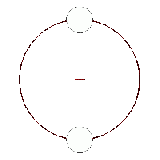Two bodies of similar mass orbiting around a common barycenter. (similar to the 90 Antiope system)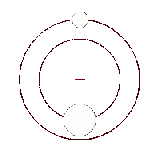Two bodies with a difference in mass orbiting around a common barycenter, as in the Pluto-Charon system.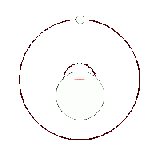Two bodies with a major difference in mass orbiting around a common barycenter (similar to the Earth-Moon system)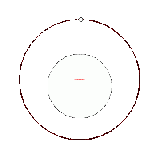Two bodies with an extreme difference in mass orbiting around a common barycenter (similar to the Sun-Earth system)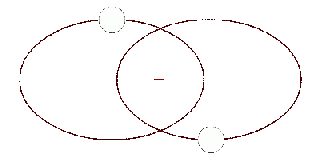Two bodies with similar mass orbiting around a common barycenter with elliptic orbits (a common situation for binary stars)

Locating the center of mass

For an arbitrary 2D physical shape

This method is useful when one wishes to find the center of gravity of a complex planar object with unknown dimensions.

 Step 1: An arbitrary 2D shape. Step 2: Suspend the shape from a location near an edge. Drop a plumb line and mark on the object. Step 3: Suspend the shape from another location not too close to the first. Drop a plumb line again and mark. The intersection of the two lines is the center of gravity.

For an L-shaped object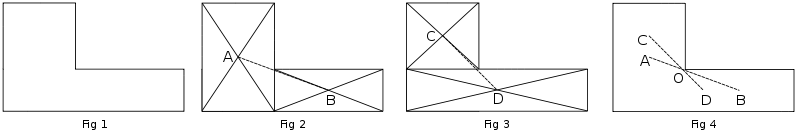1. Divide the shape into two rectangles, as shown in fig 2. Find the center of masses of these two rectangles by drawing the diagonals. Draw a line joining the center of masses. The center of mass of the shape must lie on this line AB.
2. Divide the shape into two other rectangles, as shown in fig 3. Find the center of masses of these two rectangles by drawing the diagonals. Draw a line joining the center of masses. The center of mass of the L-shape must lie on this line CD.
3. As the center of mass of the shape must lie along AB and also along CD, it is obvious that it is at the intersection of these two lines, at O. The point O might not lie inside the L-shaped object.

For a composite shape

This method is useful when you wish to find the center of gravity of an object that is easily divided into elementary shapes, whose centers of mass are easy to find. We will only be finding the center of mass in the x direction here. The same procedure may be followed to locate the center of mass in the y direction.The shape. It is easily divided into a square, triangle, and circle. Note that the circle will have negative area.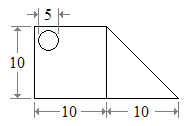From the List of centroids, we note the coordinates of the individual centroids.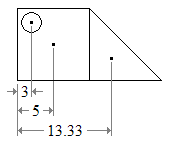From equation 1 above: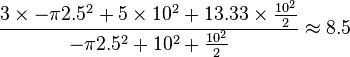$\frac{3 \times -\pi2.5^2 + 5 \times 10^2 + 13.33 \times \frac{10^2}{2}}{ -\pi2.5^2 + 10^2 + \frac{10^2}{2}} \approx 8.5$ units.

The center of mass of this figure is at a distance of 8.5 units from the left corner of the figure.

Tracing around the perimeter of a shape to locate the center of mass

A direct development of the planimeter known as an integraph, or integerometer, can be used to establish the position of the center of mass of an irregular shape. A better term is probably moment planimeter. This method can be applied to a shape with an irregular, smooth, or complex boundary where other methods are too difficult. It was regularly used by ship builders to ensure the ship would not capsize.